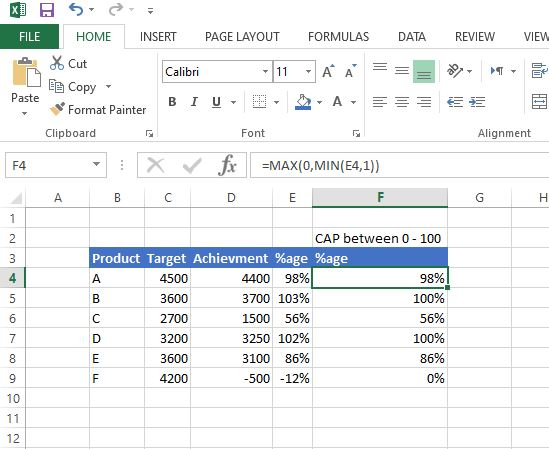# Excellent in Excel – Cap percentage between 0 and 100!

## Cap percentage between 0 and 100!

Sometimes, you need to limit values in Excel to a certain allowed range. For example, you might need to make sure that all percentages are between zero (0) and 100%. In other words, prevent negative values and values over 100%.

The classic way to do this is with a nested IF formula. But a simple and elegant alternative is to use the MIN and MAX functions. Both approaches are explained in the below:

=MAX(0,MIN(E4,1))

Summary
To limit a percentage value so that it falls between 0% and 100% you can use a formula based on the MIN and MAX functions. In the example shown, the formula in F4, copied down, is:=MAX(0,MIN(E4,1))

The result is that negative values are forced to zero, values over 1 are capped at 1, and values between 0 and 1 are unaffected.

### IF function :

As mentioned above, the IF function can also be used to solve this problem. To do this, we need two separate IF functions.

One IF forces negative values to zero:

=IF(E4<0,0,E4)

The second IF caps larger values at 1:

=IF(E4>1,1,E4)

When we nest the first IF inside the second, we have the final formula:

=IF(E4>1,1,IF(E4<0,0,E4))This is an example of a nested IF. It returns exactly the same result as the MIN and MAX formula above, but is slightly more complex and redundant. Notice, for example, the reference to E4 occurs three separate times.

The bottom line – when you need to make a choice based on smaller or larger values, the MIN and MAX functions can be a clever and elegant way to keep a formula simple.

If you need more structure, we also offer video training.

If you’ve found this tip helpful, share it.